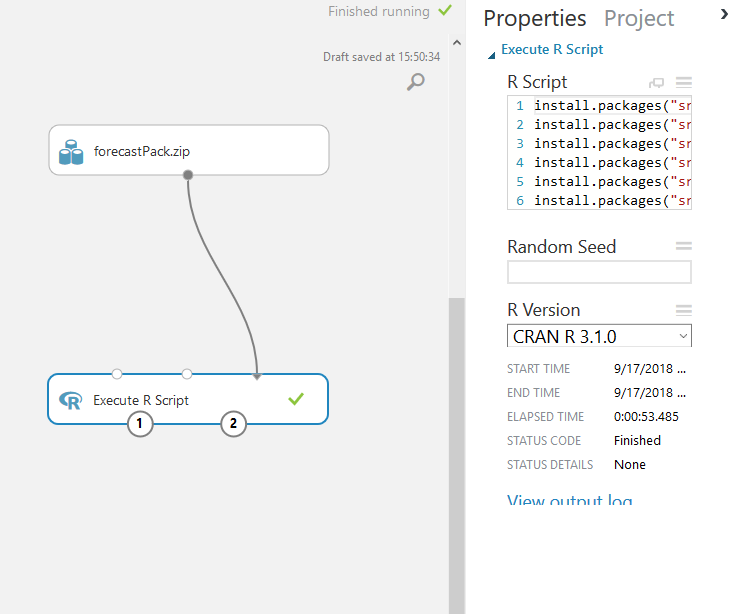top of page
Search
• Alibek Jakupov

# Customizing your Azure ML environment: The Force Awakens

Updated: Nov 19, 2021## Install R packages

There are plenty of the docs on the internet explaining how to install custom R packages in Azure ML Studio. For instance, this one gives a very detailed explanation.

However, I faced some major issues installing packages that have a long list of dependencies and making them run on Azure ML Studio. Moreover, I have also seen several stackoverflow post requesting a help in installing certain heavy packages. As the basis, let’s take this one that I responded last year, and go through all the steps I followed to cope with this limitation and to use the function tsCV on azure machine learning studio to evaluate models of forecast.

Hope this small tips and tricks will be useful for those ones who have chosen to use Azure ML studio for prototyping. Up we go!

• Install the same version of CRAN on your local machine as on the Azure ML Studio. Currently there are three kernels available in Azure ML Studio: CRAN 3.1.0, Microsoft R Open 3.2.2 and Microsoft R Open 3.4.4. CRAN (https://lnkd.in/dZpQ27M), Microsoft R Open 3.2.2 (https://lnkd.in/drWRbXN) and Microsoft R Open 3.4.4 (https://lnkd.in/dfZ9Y9Q).

• Install forecast package

1. Important: installed packages are stored in the following directory: C:\Users\<user>\Documents\R\win-library\<cran version>

2. Tip: clear this directory before installing the package – this will allow you not keep track of the dependencies during installation

• Be sure that tsCV is working locally

• Zip all the dependencies + forecast package

1. N.B Each package should be zipped separately

2. Tip: you may ran this script to zip all the folders separately

for /d %%X in (*) do "c:\Program Files\7-Zip\7z.exe" a "%%X.zip" "%%X\"
• Zip all the generated zips together and upload it to the AMLStudio

• Run the following code:

```install.packages("src/glue.zip", lib = ".", repos = NULL, verbose = TRUE)
install.packages("src/stringi.zip", lib = ".", repos = NULL, verbose = TRUE)
install.packages("src/assertthat.zip", lib = ".", repos = NULL, verbose = TRUE)
install.packages("src/fansi.zip", lib = ".", repos = NULL, verbose = TRUE)
install.packages("src/utf8.zip", lib = ".", repos = NULL, verbose = TRUE)
install.packages("src/stringr.zip", lib = ".", repos = NULL, verbose = TRUE)
install.packages("src/labeling.zip", lib = ".", repos = NULL, verbose = TRUE)
install.packages("src/munsell.zip", lib = ".", repos = NULL, verbose = TRUE)
install.packages("src/R6.zip", lib = ".", repos = NULL, verbose = TRUE)
install.packages("src/RColorBrewer.zip", lib = ".", repos = NULL, verbose = TRUE)
install.packages("src/cli.zip", lib = ".", repos = NULL, verbose = TRUE)
install.packages("src/crayon.zip", lib = ".", repos = NULL, verbose = TRUE)
install.packages("src/pillar.zip", lib = ".", repos = NULL, verbose = TRUE)
install.packages("src/xts.zip", lib = ".", repos = NULL, verbose = TRUE)
install.packages("src/TTR.zip", lib = ".", repos = NULL, verbose = TRUE)
install.packages("src/curl.zip", lib = ".", repos = NULL, verbose = TRUE)
install.packages("src/digest.zip", lib = ".", repos = NULL, verbose = TRUE)
install.packages("src/gtable.zip", lib = ".", repos = NULL, verbose = TRUE)
install.packages("src/lazyeval.zip", lib = ".", repos = NULL, verbose = TRUE)
install.packages("src/plyr.zip", lib = ".", repos = NULL, verbose = TRUE)
install.packages("src/reshape2.zip", lib = ".", repos = NULL, verbose = TRUE)
install.packages("src/rlang.zip", lib = ".", repos = NULL, verbose = TRUE)
install.packages("src/scales.zip", lib = ".", repos = NULL, verbose = TRUE)
install.packages("src/tibble.zip", lib = ".", repos = NULL, verbose = TRUE)
install.packages("src/viridisLite.zip", lib = ".", repos = NULL, verbose = TRUE)
install.packages("src/withr.zip", lib = ".", repos = NULL, verbose = TRUE)
install.packages("src/quadprog.zip", lib = ".", repos = NULL, verbose = TRUE)
install.packages("src/quantmod.zip", lib = ".", repos = NULL, verbose = TRUE)
install.packages("src/colorspace.zip", lib = ".", repos = NULL, verbose = TRUE)
install.packages("src/fracdiff.zip", lib = ".", repos = NULL, verbose = TRUE)
install.packages("src/ggplot2.zip", lib = ".", repos = NULL, verbose = TRUE)
install.packages("src/lmtest.zip", lib = ".", repos = NULL, verbose = TRUE)
install.packages("src/magrittr.zip", lib = ".", repos = NULL, verbose = TRUE)
install.packages("src/Rcpp.zip", lib = ".", repos = NULL, verbose = TRUE)
install.packages("src/timeDate.zip", lib = ".", repos = NULL, verbose = TRUE)
install.packages("src/tseries.zip", lib = ".", repos = NULL, verbose = TRUE)
install.packages("src/urca.zip", lib = ".", repos = NULL, verbose = TRUE)
install.packages("src/uroot.zip", lib = ".", repos = NULL, verbose = TRUE)
install.packages("src/zoo.zip", lib = ".", repos = NULL, verbose = TRUE)
install.packages("src/RcppArmadillo.zip", lib = ".", repos = NULL, verbose = TRUE)
install.packages("src/forecast.zip", lib = ".", repos = NULL, verbose = TRUE)

library(forecast, lib.loc=".", verbose=TRUE)
far2 <- function(x, h){forecast(Arima(x, order=c(2,0,0)), h=h)}
e <- tsCV(lynx, far2, h=1) ```

• Important: how I obtained this order? I simply copied the installation log and added install.packages in front of each package

• Important: you don’t really need to install all the dependencies, as only few of them are not pre-installed. So, the logic is quite simple: you comment all the lines and leave only the last one. After compilation Azure will send you error that package X is missing. Just uncomment the line containing the package and so on and so forth.Here is the screen shot of my experiment.

And, of course, here is the zip package with forecast library: enjoy!

Hope this was useful. Together we can make everything better.